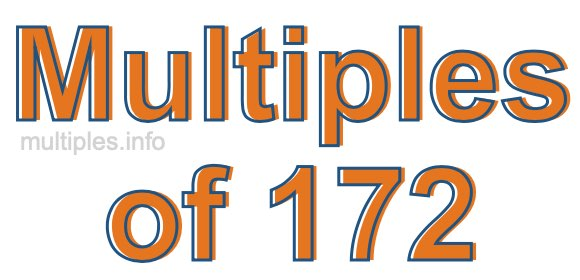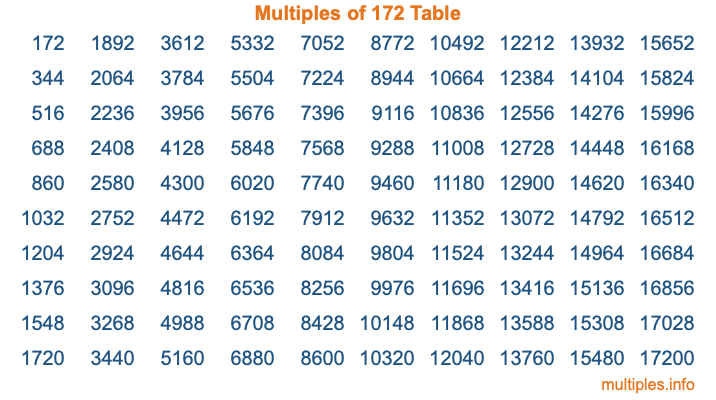Multiples of 172Welcome to the Multiples of 172 page. Here we will first teach you everything you will ever need to know about the multiples of 172, and then give you a study guide summary of everything we taught you to make sure you remember it all. Use this page to look up facts and learn information about the multiples of 172. This page will make you a multiples of one hundred seventy-two expert!

Definition of Multiples of 172
Multiples of 172 are all the numbers that when divided by 172 equal an integer. Each of the multiples of 172 are called a multiple. A multiple of 172 is created by multiplying 172 by an integer.

Therefore, to create a list of multiples of 172, you start with 1 multiplied by 172, then 2 multiplied by 172, then 3 multiplied by 172, and so on for as long as you want. Thus, the list of the first five multiples of 172 is 172, 344, 516, 688, and 860. To see a larger list of multiples of 172, see the printable image of Multiples of 172 further down on this page. We also have a category where you can choose any nth multiple of 172.

Multiples of 172 Checker
The Multiples of 172 Checker below checks to see if any number of your choice is a multiple of 172. In other words, it checks to see if there is any number (integer) that when multiplied by 172 will equal your number. To do that, we divide your number by 172. If the the quotient is an integer, then your number is a multiple of 172.

Is  a multiple of 172?

Least Common Multiple of 172 and ...
A Least Common Multiple (LCM) is the lowest multiple that two or more numbers have in common. This is also called the smallest common multiple or lowest common multiple and is useful to know when you are adding our subtracting fractions. Enter one or more numbers below (172 is already entered) to find the LCM.

Check out our LCM Calculator if you need more details about the Least Common Multiple or if you need the LCM for different numbers for adding and subtraction fractions.

nth Multiple of 172
As we stated above, 172 is the first multiple of 172, 344 is the second multiple of 172, 516 is the third multiple of 172, and so on. Enter a number below to find the nth multiple of 172.

th multiple of 172

Multiples of 172 vs Factors of 172
172 is a multiple of 172 and a factor of 172, but that is where the similarities end. All postive multiples of 172 are 172 or greater than 172. All positive factors of 172 are 172 or less than 172.

Below is the beginning list of multiples of 172 and the factors of 172 so you can compare:

Multiples of 172: 172, 344, 516, 688, 860, etc.

Factors of 172: 1, 2, 4, 43, 86, 172

As you can see, the multiples of 172 are all the numbers that you can divide by 172 to get a whole number. The factors of 172, on the other hand, are all the whole numbers that you can multiply by another whole number to get 172.

It's also interesting to note that if a number (x) is a factor of 172, then 172 will also be a multiple of that number (x).

Multiples of 172 vs Divisors of 172
The divisors of 172 are all the integers that 172 can be divided by evenly. Below is a list of the divisors of 172.

Divisors of 172: 1, 2, 4, 43, 86, 172

The interesting thing to note here is that if you take any multiple of 172 and divide it by a divisor of 172, you will see that the quotient is an integer.

Multiples of 172 Table
Below is an image of the first 100 multiples of 172 in a table. The table is in chronological order, column by column. The first column has the first ten multiples of 172, the second column has the next ten multiples of 172, and so on.The Multiples of 172 Table is also referred to as the 172 Times Table or Times Table of 172. You are welcome to print out our table for your studies.

Negative Multiples of 172
Although not often discussed or needed in math, it is worth mentioning that you can make a list of negative multiples of 172 by multiplying 172 by -1, then by -2, then by -3, and so on, to get the following list of negative multiples of 172:

-172, -344, -516, -688, -860, etc.

Multiples of 172 Summary
Below is a summary of important Multiples of 172 facts that we have discussed on this page. To retain the knowledge on this page, we recommend that you read through the summary and explain to yourself or a study partner why they hold true.

There are an infinite number of multiples of 172.

A multiple of 172 divided by 172 will equal a whole number.

172 divided by a factor of 172 equals a divisor of 172.

The nth multiple of 172 is n times 172.

The largest factor of 172 is equal to the first positive multiple of 172.

172 is a multiple of every factor of 172.

172 is a multiple of 172.

A multiple of 172 divided by a divisor of 172 equals an integer.

172 divided by a divisor of 172 equals a factor of 172.

Any integer times 172 will equal a multiple of 172.

Multiples of a Number
Here you can get the multiples of another number, all with the same attention to detail as we did for multiples of 172 on this page.

Multiples of
Multiples of 173
Did you find our page about multiples of one hundred seventy-two educational? Do you want more knowledge? Check out the multiples of the next number on our list!